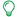# QlikView Documents

QlikView documentation and resources.

Announcements
Read about the latest Qlik Community enhancements on the Community News blog!

# Ignore all selections except some specific fields using Set AnalysisNot applicable

## Ignore all selections except some specific fields using Set Analysis

Hello.

As far as I was designing apps for QV - I've found a great need in some charts to ignore all possible selections except some specific fields.

Exploring possible ways to do so - the first my decision was to use dollar-sign expression inside my Set Analysis which could make an exception like next:
(Let's imagine that in my scheme there are fields [Stock], [Manager], [Date], [City], [Client] and [Amount])

`sum({1<[Stock]={'Stock 1','Stock 3'}>} Amount)`

If I selected only 'Stock 1' and 'Stock 3' in field [Stock] - and this way has shown it's profit - you can get it using this expression:

`sum({1<[Stock]=\$(=if(GetSelectedCount([Stock], true( ))>0,'{' & chr(39) & GetFieldSelections([Stock],chr(39) & ',' & chr(39),GetSelectedCount([Stock], true( ))) & chr(39) & '}', ''))>} Amount)`

If you need two, three, or more fields - you can simply add such code for every field into Set Analysis:

`sum({1<[Stock]=\$(=if(GetSelectedCount([Stock], true( ))>0,'{' & chr(39) & GetFieldSelections([Stock],chr(39) & ',' & chr(39),GetSelectedCount([Stock], true( ))) & chr(39) & '}', '')),[Manager]=\$(=if(GetSelectedCount([Manager], true( ))>0,'{' & chr(39) & GetFieldSelections([Manager],chr(39) & ',' & chr(39),GetSelectedCount([Manager], true( ))) & chr(39) & '}', ''))>} Amount)`

But what if you have a field containing hundreds of records? Can you imagine what will be the length of your expression? That's why I started to discover another way to do that. I've thought - what if I use not "1" Set, but "\$" Set in my expression and write in it all the fields that must be ignored, something like that:

`sum({\$<[Date]=,[City]=,[Client]=,[Amount]=>} Amount)`

So, using such expression - I get that all the fields except for [Stock] and [Manager] are ignored, but how car I make it automatic? Sure using our lovely dollar-sign expressions and system field \$Field:

`sum({\$<[\$(=Concat({1<\$Field-={'Stock','Manager'}>}distinct \$Field,']=,[')&']=')>} Amount)`

where inside the set analysis of Concat function you can insert fields that you are interested in (by the way - there you can insert even tables using system field \$Table and others).

Labels (1)
• ### App DevelopmentChampion III
Clever. I'll need to remember that for cases such as ignoring all date fields from the calendar except the one(s) I'm actually doing set analysis on.Not applicable

I think I don't understand the last expression...

How could I use this to get this setup:

I have a chart (graph) with a dimension lets say Category and Date (MMM-YYYY). What I'm trying to do is limit the Date-selectionbox to 1 selectable value that represents 1 month of 1 year. The graph then needs to show the selected month together with 3 previous months. As measure lets say I just want to count the A-values so something like Sum(if(Value='A',1,0)).

I was trying to make this setup by using a set expression

Sum( { 1 < Date={ " >=\$(=AddMonths(Date,-3)) <\$(=AddMonths(Date,1)) " } > } if(Value='A',1,0) )

The Graph works for the date, it show only the period that I need. But when I make a subselection of Categories, it keeps showing the full result, so all possible Categories.

Any ideas?Not applicable

If you need a graph to react only on selections in fields Date and Category - you should use expression like:

sum({\$<\$(=Concat({1<\$Field-={'Date','Category'}>}distinct '['&\$Field&']=',','))>} if(Value='A',1,0))

also if you need to select some Dates and have possibility to select ranges inside this range of dates - I'll advise you to add into Set Analisys union with correct modified "1" Set^

and if you need to have static range of dates independent to your selection:Not applicable

Great post! I just used this for a use case where I needed to ignore all of the fields in a table and used the \$Table system field. Using your example it would be:

`sum({\$<[\$(=Concat({1<\$Table={'Table1'}>}distinct \$Field,']=,[')&']=')>} Amount)`Not applicable

I was looking for a solution for this problem too. I found this post also very handy:

As far as I can see, it is another solution for the same problem.Master II

ExcellentNot applicable

Very useful! Also combinations are possible:

count({<\$(=concat({<\$Table-={"ADDRESS"}, \$Field-={"CUSTOMER*"}>} '[' & \$Field & ']=',','))>} ID)Partner

This is a really, really useful.Partner

Thanks to your wonderful posting I have been able to put together the following "ratio" - as I select a year/month combination the numbers that are coming up are perfect. As I select my month/year combo (both these are in Calendar table) the figures Min(DateNo) and Max(DateNo) represent the boundaries of the month selected (1/11/2013 and 30/11/2013 for example in the case of Nov 2013).

I have tried to PLOT these in a line graph however and I am getting incorrect results - the dimension is "MonthYear" ("Nov-2013") which is in my "Calendar" table. "DateNo" is also a field in my calendar table and contains the day number (e.g.  41579 for 1/11/2013)

The expression I use is:

= num(count ({\$} DISTINCT TID)

/

sum({\$<[\$(=Concat({1<\$Field-={'VendorName','ModelName''}>}distinct \$Field,']=,[')&']='),

[ProdOUTNumeric] = {'>=\$(=Min(DateNo))'},

[ProdINNumeric] = {'<=\$(=Max(DateNo))'} >} TransRecNo),  '##.##%')

To help me debug this I created 2 text boxes to see what values the numerator and denominator from above calculate to and they are correct when I select a particular month but when plotted it gives me incorrect figures. Am I missing something?

I would really appreciate some help

AlexisEnthusiast

Excellent. It helps me lot to resolve my problem. Thank you very much.

Version history
Revision #:
1 of 1
Last update:
‎2011-01-26 06:06 AM
Updated by: